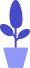# Python numbers and math operations

Estimated reading: 3 minutes 103 views

Numbers in Python can be divided into integers, decimals, and complex numbers, all of which can participate in mathematical operations. This section will focus on integers and decimals.

## Numbers and Math Operations

Python refers to positive and negative integers without a decimal point as integers . In Python, we can perform basic mathematical operations on integers such as addition, subtraction, multiplication, and division. To do these operations, we use the arithmetic operators +, −, * and /. Let’s look at a more complicated example. What is 12345 plus 56789?

``````>>> 12345+56789
69134``````

Mental arithmetic is not easy, but Python can calculate it effortlessly.

We can also add multiple numbers together at once:

``````>>> 22+33+44
99``````

Python can also do subtraction:

``````>>> 1000-17
983``````

You can also use asterisks `*`for multiplication:

``````>>> 123*456
56088``````

Use slashes `/`for division:

``````>>> 12345/25
493.8``````

We can also combine these simple operations into a more complex calculation:

``````>>> 987+47*6-852/3
985.0``````

According to the rules of mathematics, multiplication and division are always done before addition and subtraction, and Python also follows this rule. Figure 1 shows the order in which Python performs computations:

• First, perform multiplication, 47*6 gets 282 (represented in red font);
• Then, the division operation is performed, 852/3 gets 284.0 (indicated in green font);
• Next, perform an addition operation, 987+282 get 1269 (indicated in blue font);
• The subtraction is finally calculated, 1269−284.0 gives 985.0 (in purple), which is the final result.

Figure 1: Python execution order of computations

Note that here our result is a float with decimals.

``In Python 3, /the result of division contains decimals. If you only want to take integers, you need to use them //; in Python 2, /the result of division will take integers.``

#### The role of parentheses

What if you want to perform addition and subtraction before multiplication and division?

For example, suppose you have two good friends, and now you have 9 apples. You want to divide the apples equally between yourself and your friends. What should you do? You have to divide the number of apples by the number of people who divided the apples.

Here is an attempt:

``````>>> 9/1+2
11.0``````

This result is obviously wrong. When you only have 9 apples, you can’t give each person 11 apples. The problem is that Python does the division before it does the addition. It divides 9 by 1 (which equals 9), and then adds 2 to get 11.

To fix this so that Python does the addition first, we need to use parentheses:

``````>>> 9/(1+2)
3.0``````

That’s right, 3 apples per person. The parentheses force Python to calculate 1 plus 2, and then divide 9 by 3.

## floating point number

Numbers with a decimal point are called floating point numbers . Examples of floating point numbers are as follows:

``````>>> 0.1+0.1
0.2
>>> 4*0.2
0.8
>>> 4.8/2
2.4
>>> 2-0.8
1.2
>>> 9/3
3.0``````

It should be noted that sometimes, the number of decimal places contained in the operation result may be uncertain:

``````>>> 0.2+0.1
0.30000000000000004
>>> 4.8/0.4
11.999999999999998``````

We see that 0.2+0.1 is not equal to 0.3, but equal to 0.30000000000000004. This is not a problem with Python, all binary-based floating point numbers have this problem, it’s a problem with the computer itself.

Python will try to find a way to represent the result as accurately as possible, but given the way numbers are represented internally by computers, this is difficult to do in some cases.